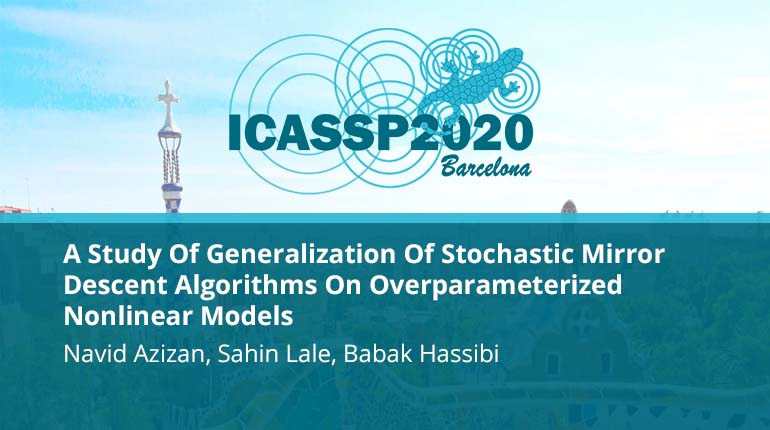# A Study Of Generalization Of Stochastic Mirror Descent Algorithms On Overparameterized Nonlinear Models

Collection:We study the convergence, the implicit regularization and the generalization of stochastic mirror descent (SMD) algorithms in overparameterized nonlinear models, where the number of model parameters exceeds the number of training data points. Due to overp
• IEEE MemberUS \$11.00
• Society MemberUS \$0.00
• IEEE Student MemberUS \$11.00
• Non-IEEE MemberUS \$15.00
Purchase

## A Study Of Generalization Of Stochastic Mirror Descent Algorithms On Overparameterized Nonlinear Models

We study the convergence, the implicit regularization and the generalization of stochastic mirror descent (SMD) algorithms in overparameterized nonlinear models, where the number of model parameters exceeds the number of training data points. Due to overp